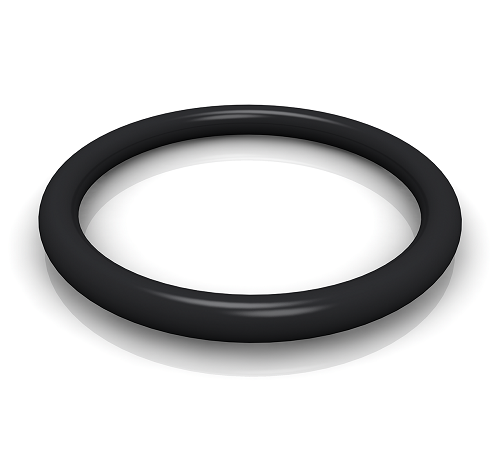O-rings are the most widely-used seals because they are efficient, economical, and can be used in a wide variety of static and dynamic applications. In order to get the most out of an O-ring, it is critical that its dimensions and specifications meet part requirements. Sometimes, this can be difficult if a part is metric and the only available seals have Imperial dimensions or vice versa. Still, this is not an insurmountable obstacle if an engineer knows the conversions involved. Here are some common seal units and the conversions between metric and Imperial units for them.

There are several specifications that are essential to selecting the correct O-ring. The inside diameter, outside diameter and cross section define the dimensions of an O-ring. The inside and outside diameters are theAn O-ring. Source: Trelleborg Sealing Solutionsdistances from the center to the O-ring’s inside and outside surfaces, respectively. The cross section is the difference between the outer and inner diameters. All three dimensions are typically given in millimeters (mm) for metric O-rings and inches (in) for Imperial O-rings.

One inch is equal to 25.4 mm, so when converting from an Imperial dimension to a metric dimension, simply multiply by 25.4 mm/in. In order to convert from metric to Imperial dimensions, multiply by the inverse of 25.4 mm/in, which is approximately 0.0394 in/mm. Imperial specifications are sometimes given as fractions (such as 1/3”) where the quote symbol after the fraction represents the Imperial unit inch. In these cases, the first step is to always convert the fraction to a decimal, then perform the unit conversion. Thus, for example, 1/3” = ~0.33 in = 0.33 in x 25.4 mm/in = ~8.5 mm.

Another important O-ring specification is the maximum pressure rating. Generally, this tends to be expressed in megapascals (MPa) for metric O-rings. Imperial O-rings usually express this specification in units of pound-force per square inch (psi). One MPa is equal to approximately 145 psi. Therefore, to convert the required maximum pressure for an O-ring to Imperial units, multiply by 145 psi/MPa. To convert an Imperial maximum pressure to MPa, multiply by the inverse, equal to ~.007 MPa/psi. Occasionally, rather than MPa, maximum pressure will be given in bar. A bar is simply one-tenth of an MPa, so the conversion here is simple: 1 bar = 0.1 MPa = 14.5 psi.

Operating temperature range is another important specification that helps one understand whether an O-ring can withstand the environmental challenges of its application. It is therefore very important that a conversion is done correctly when comparing metric and Imperial units. Metric O-rings have temperature ranges given in degrees Celsius. Imperial O-rings have operating temperature ranges given in degrees Fahrenheit.

To convert from Celsius to Fahrenheit, use the following formula:

T(°F) = T(°C) × 1.8 + 32

To convert from Fahrenheit to Celsius, use the following formula:

T(°C) = (T(°F) - 32) / 1.8

The specifications of inner diameter, outer diameter, cross section, maximum pressure and operating temperature range are critical to accurately identifying the correct O-ring for an application. Consequently, it is imperative, when confronted by metric or Imperial units for these O-rings or their applications, that an engineer is able to convert between the two unit systems for those specifications. Although the metric and imperial units don’t have to exactly agree, it is a good idea to try to get them as close as possible for optimal O-ring performance and part life.